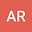•In this report we prove that the hypothesis on the memory term $g$ in \cite% {WenjunYunSun} can be modified to be $g^{\prime }(t)\leq -\zeta (t)g^{p}(t)$% , $t\geq 0,$ $1\leq p<\frac{3}{2}$ where $\zeta (t)$ provides% \begin{equation*} \zeta \left( 0\right) >0,\text{ }\zeta ^{\prime }(t)\leq 0,\text{ }% \int_{0}^{\infty }\zeta \left( s\right) ds=+\infty . \end{equation*}% So the optimal decay results are extended.